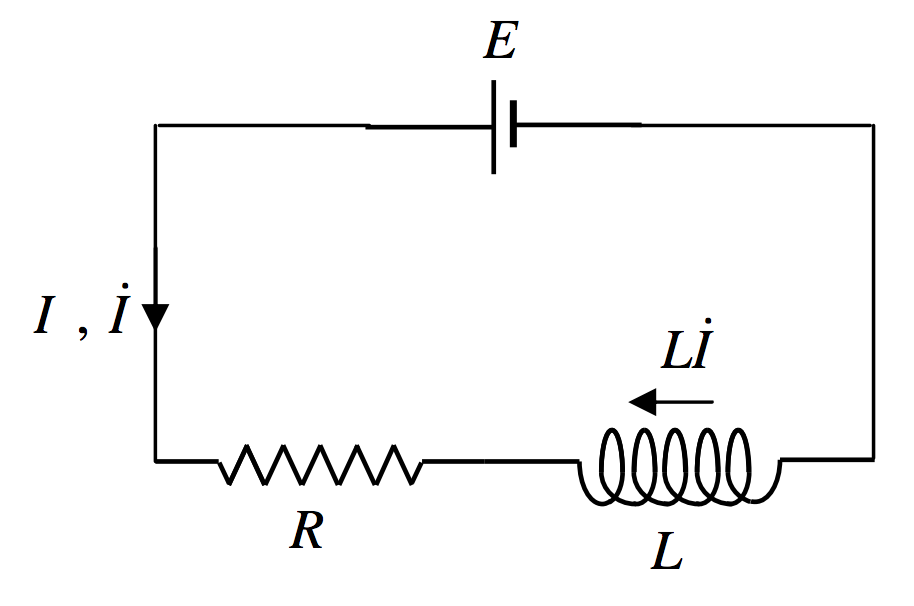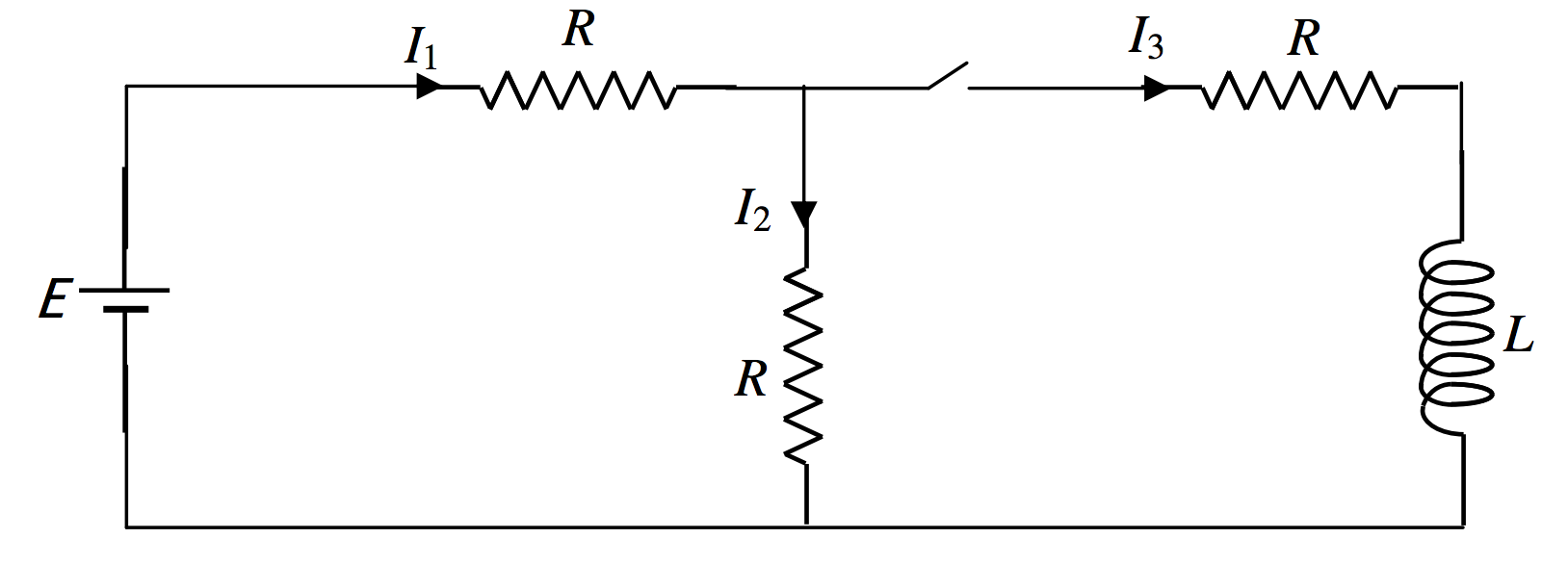$$\require{cancel}$$

# 10.12: Growth of Current in a Circuit Containing Inductance

•• Contributed by Jeremy Tatum
• Emeritus Professor (Physics & Astronomy) at University of Victoria

It will have occurred to you that if the growth of current in a coil results in a back EMF which opposes the increase of current, current cannot change instantaneously in a circuit that contains inductance. This is correct. (Recall also that the potential difference in a circuit cannot change instantaneously in a circuit containing capacitance. Come to think of it, it is hardly possible for the capacitance or inductance of any circuit to be exactly zero; any real circuit must have some capacitance and inductance, even if very small.)

Consider the circuit of Figure X.9. A battery of EMF $$E$$ is in series with a resistance and an inductance. (A coil or solenoid or any inductor in general will have both inductance and resistance, so the $$R$$ and the $$L$$ in the Figure may belong to one single item.) We have to be very careful about signs in what follows.$$\text{FIGURE X.9}$$

When the circuit is closed (by a switch, for example) a current flows in the direction shown. by an arrow, which also indicates the direction of the increase of current. An EMF $$L\dot I$$ is induced in the opposite direction to $$\dot I$$. Thus, Ohm's law, or, if your prefer, Kirchhoff's second rule, applied to the circuit (watch the signs carefully) is

$E=IR -L \dot I=0.\label{10.12.1}$

Hence:

$\label{10.12.2}\int_0^I \frac{dI}{\frac{E}{R}-I}=\frac{R}{L}\,dt.$

Warning: Some people find an almost irresistible urge to write this as $$\int_0^I \frac{dI}{I-\frac{E}{R}}=-\frac{R}{L}\,dt$$.

Don't!

You can anticipate that the left hand side is going to be a logarithm, so make sure that the denominator is positive. You may recall a similar warning when we were charging and discharging a capacitor through a resistance.

Integration of equation \ref{10.12.2} results in the following equation for the growth of the current with time:

$I=\frac{E}{R}\left (1-e^{-(R/L)t}\right ) .\label{10.12.3}$

Thus the current asymptotically approaches its ultimate value of $$E/R$$, reaching 63% (i.e. $$1-e^{-1}$$ ) of its ultimate value in a time $$L/R$$. In Figure X.10, the current is shown in units of $$E/R$$, and the time in units of $$L/R$$. You should check that $$L/R$$, which is called the time constant of the circuit, has the dimensions of time.$$\text{FIGURE X.10}$$

Here is a problem that will give practice in sending a current through an inductor, applying Kirchhoff’s rules, and solving differential equations. There is a similar problem involving a capacitor, in Chapter 5, Section 5.19.In the above circuit, while the switch is open, $$I_1 = I_2 = E/(2 R)\text{ and }I_3 = 0$$. Long after the switch is closed and steady currents have been reached, $$I_1$$ will be $$2E /(3R)$$, and $$I_2 \text{ and }I_3$$ will each be $$E /(3R)$$. But we want to investigate what happens in the brief moment while the current is changing.

We apply Kirchhoff’s rules:

$\label{10.12.4}E=I_1R+I_2R$

$\label{10.12.5}I_3R+L\dot I_3 -I_2R=0$

$\label{10.12.6}I_1=I_2+I_3,$

[Getting the sign of $$L\dot I_3$$ right in equation \ref{10.12.5} is important. Think of the inductor as a battery of EMF $$L\dot I_3$$ oriented like this:.]

Eliminate $$I_1 \text{ and }I_2$$ to get a single equation in $$I_3$$.

$\label{10.12.7}\frac{dI_3}{dt}+\frac{3R}{2L}I_3 =\frac{E}{2L}.$

This is of the form $$\frac{dy}{dx}+ay=b$$, and those experienced with differential equations will have no difficulty in arriving at the solution

$\label{10.12.8}I_3 = \frac{E}{3R}+Ae^{\frac{3Rt}{2L}}.$

With the initial condition that $$I_3 = 0 \text{ when }t = 0$$, this becomes

$\label{10.12.9}I_3=\frac{E}{3R}\left ( 1-e^{-\frac{3Rt}{2L}}\right )$

The other currents are found from Kirchhoff’s rules (equations \ref{10.12.4}-6). I make them:

$\label{10.12.10}I_2 = \frac{E}{3R}\left ( 1+\frac{1}{2}e^{-\frac{3Rt}{2L}}\right )$

$\label{10.12.11}I_1 = \frac{E}{3R}\left ( 2-\frac{1}{2}e^{-\frac{3Rt}{2L}}\right )$

Thus $$I_1$$ goes from initially $$\frac{E}{2R}\text{ to finally }\frac{2E}{3R}$$.

$$I_2$$ goes from initially $$\frac{E}{2R}\text{ to finally }\frac{E}{3R}$$.

$$I_3$$ goes from initially zero to finally $$\frac{E}{3R}$$.

Here are graphs of the currents (in units of $$E/R$$) as a function of time (in units of $$2L /(3R)$$).